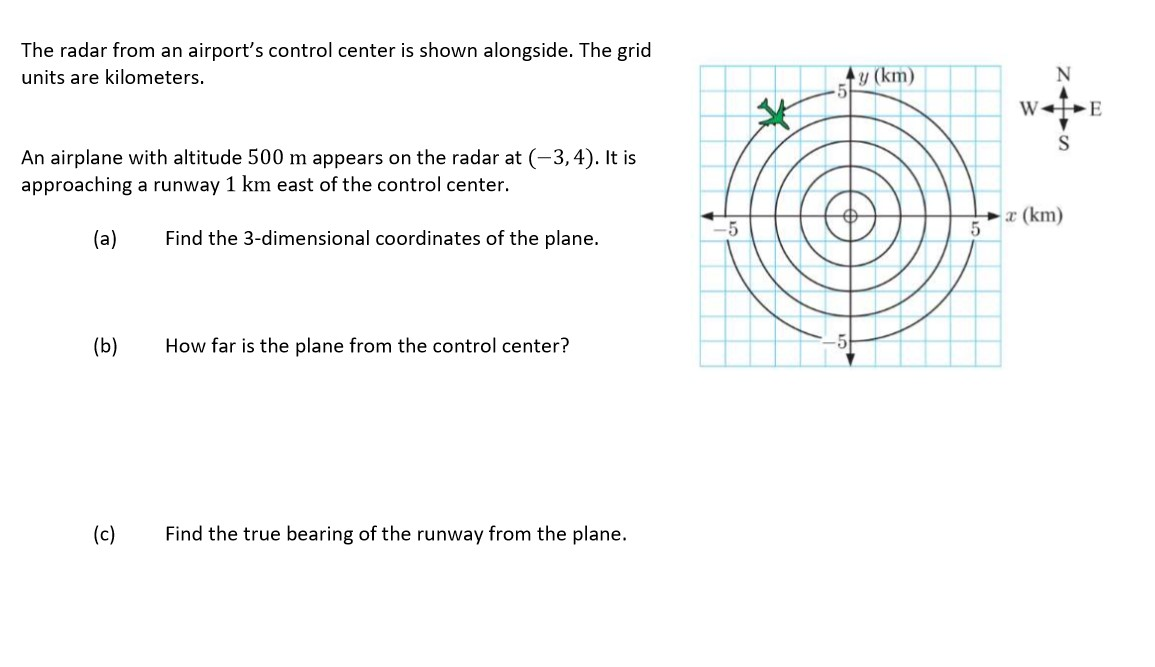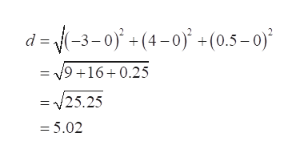# The radar from an airport's control center is shown alongside. The gridy (km)units are kilometers.An airplane with altitude 500 m appears on the radar at (-3,4). It isapproaching a runway 1 km east of the control center.a (km)Find the 3-dimensional coordinates of the plane.(a)(b)How far is the plane from the control center?Find the true bearing of the runway from the plane.(c)

Question
89 viewshelp_outlineImage TranscriptioncloseThe radar from an airport's control center is shown alongside. The grid y (km) units are kilometers. An airplane with altitude 500 m appears on the radar at (-3,4). It is approaching a runway 1 km east of the control center. a (km) Find the 3-dimensional coordinates of the plane. (a) (b) How far is the plane from the control center? Find the true bearing of the runway from the plane. (c) fullscreen
check_circle

Step 1

Bearing angle is the measure of angle in clockwise direction from northline.

Distance formula for the figure in 3D between two point (a,b,c) and (x,y,z) is given as,

Step 2
1. a) Convert 500 m to km

1m= 0.001km

500m=0.5Km

0.5Km would be the z-coordinate

Thus, the 3D coordinate of the plane will be

Step 3

Distance between origin (0,0,0) and ...help_outlineImage Transcriptionclosed = (-3-0) +(4-0)* +(0.5 – 0)² = 19 +16+0.25 = V25.25 = 5.02 fullscreen

### Want to see the full answer?

See Solution

#### Want to see this answer and more?

Solutions are written by subject experts who are available 24/7. Questions are typically answered within 1 hour.*

See Solution
*Response times may vary by subject and question.
Tagged in